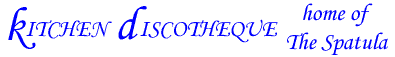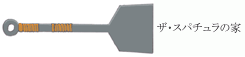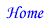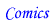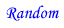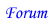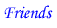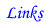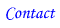The Impact of the Supernova in Uchuu no StellviaHow much destruction would the first or second wave in the anime Uchuu no Stellvia (Stellvia of the Universe) actually cause? This may seem like a frivolous activity, but one cannot deny the central role that this phenomenon plays in this series. Using simple algebra and some logical thinking, we can determine if we should worry about something like this. Before we actually do any calculations, we first need to establish a couple of assumptions based on real facts that can be found via a quick web search. ASSUMPTIONS The star that goes supernova, Beta Hydri, is seemingly based on an actual star called Hydrus Beta, which is 20 light years (lt-yrs) away from earth (the actual distance is 24 lt-yrs, which was corrected recently in the astronomical data books, but we will go with what they have in the anime since either number would give answers that are within an order of magnitude of each other). There are no obstacles in the path of the explosion (e.g., planets, asteroid belts, other stars, black holes, etc.). The energy of a typical supernova explosion is 10^44 Joules (J). Useful Constants: pi = 3.141592654 radians 1 year = 3.15576x10^7 s The speed of light (c) = 2.998x10^5 km/s 1 light year (lt-yr) = 9.46096848x10^12 km Radius of the Earth = 6.371x10^3 km CALCULATIONS One of the cool things about equations is that you don't need to have the correct numbers in order to set up a proper equation. Even if the information in the previous section was wrong, we can simply plug in the correct ones later. (Note: I will leave on the extra digits and round at the end.) Something we need to consider is the fact that not all of the shockwave would hit the earth. Picture a balloon expanding next to a golf ball. Only a certain amount of the surface would actually touch the golf ball. This will give us an idea of how much of the total energy we would need to consider in any subsequent calculations relating to the explosion. If we model the explosion as a spherical shell with negligible thickness that expands outward from the original location of Beta Hydri, we use the typical formula for the surface area of a sphere: (Eq. 1)   Surface Area of a Sphere = 4*pi*R^2 where R = Distance to Earth from Beta Hydri = 20 lt-yrs It is more convenient to use kilometers so we will perform the following conversion: (Eq. 2)   (20 lt-yrs)*[9.46096848x10^12 (km/lt-yr)] = (An. 1)   1.892193696x10^14 km Now, if we use the values from above in (Eq. 1), we obtain the following: (An. 2)   Total Surface Area of Explosion = 4.499259544x10^29 km^2 Next, we calculate how much of the surface area will actually hit the earth. Again, if we use the balloon and golf ball model above, only the surface of the earth that is facing the explosion would be affected. We model this by assuming that the earth is a disk. The earth may be a hemisphere, but the amount of flux is equivalent to that of a disk - if you don't believe me, go ahead and do the integral. If we use the typical formula to calculate the area of a circle (Eq. 3)   Area of a Circle = pi*Re^2 where Re = Radius of the Earth = 6.371x10^3 km we get (An. 3)   Area of Earth Disk = 1.27516118x10^8 km^2 Finally, we can calculate the percentage of the total energy that would hit the earth using (An. 3) & (An. 2) in the following equation: (Eq. 4)   (Area of E.D.)/(Total S.A. of Explosion) = Fraction of Total S.A. of Explosion Hitting the Earth we obtain the following value: (An. 4)   Fraction of Total = 2.83415786x10^-22 I don't know about you, but that seems like a pretty small number to me; however, this number has no meaning by itself, so let's put this in the context of the anime. There are two shockwaves that hit the earth, so there must be some distribution of the energy between the two. Right now, let's assume that ALL (100%) of the energy of the supernova explosion went to the first shockwave. Then (Eq. 5)   (Fraction of Total S.A.)*(Energy of Supernova) = (An. 5) Fraction of Supernova Energy Hitting Earth = 2.8x10^22 J To put this in perspective, the Atomic Bomb used on Hiroshima released approximately 10^14 J, so the energy of the first shockwave (an Electromagnetic pulse) would be equivalent to around 280 million of those bombs. Ouch. Another way to look at it is if we assume that the incident solar power hitting the earth is approximately 10^17 Watts, then the first shockwave is around 1 million times worse. Anyone unlucky enough to be standing outside on the part of the earth that is facing the supernova at the time would be burnt to a crisp - unless they had some SPF 1,000,000 suntan lotion. Can we say Robocop? Even if this energy were divided evenly between the first and second shockwave, the amount of energy would still be significant. Now, we could calculate the destructive force of the debris from the expired star (during a supernova, heavier elements than helium are created), but it is already apparent that it would carry quite a bit of energy. Preparation for a second shockwave is only logical. I want to equate the destruction to the popular "planet-killing asteroids" but I need to look up some more information. Look for a future update! ^_^ CONCLUSION Apparently, the producers of Stellvia did their homework. If a relatively close star were to supernova, then people on Earth would be pretty screwed. Needless to say, the results would be much worse if a closer star like Proxima Centauri were to supernova. I applaud their efforts in putting some thought into making an integral part of the story seem reasonable in terms of the physical consequences. Other scientific aspects of the story are questionable, but I'm willing to let it slide... As a final note, I, like any other kitchen utensil, am prone to error, so if you notice anything wrong with any of the above, let me know so I can fix it. Copyright © for images goes to the respective owners. Page content copyright © 2003-2008 by the site owner. All rights reserved.